The PHREG Procedure
 Failure Time Distribution

Let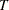be a nonnegative random variable representing the failure time of an individual from a homogeneous population. The survival distribution function (also known as the survivor function) ofis written as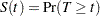A mathematically equivalent way of specifying the distribution ofis through its hazard function. The hazard function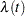specifies the instantaneous failure rate at. Ifis a continuous random variable,is expressed as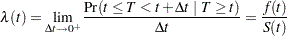where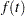is the probability density function of. Ifis discrete with masses at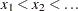, thenis given by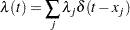where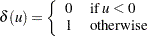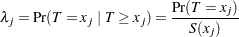for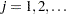Previous Page | Next Page | Top of Page